GeeksforGeeks App
Open AppBrowser
Continue

# Median of two sorted arrays with different sizes in O(log(min(n, m)))

Given two sorted arrays, a[] and b[], the task is to find the median of these sorted arrays, in O(log(min(n, m)), when n is the number of elements in the first array, and m is the number of elements in the second array.

Prerequisite : Median of two different sized sorted arrays.

Examples :

```Input : ar1[] = {-5, 3, 6, 12, 15}
ar2[] = {-12, -10, -6, -3, 4, 10}
The merged array is :
ar3[] = {-12, -10, -6, -5 , -3,
3, 4, 6, 10, 12, 15}
Output : The median is 3.

Input : ar1[] = {2, 3, 5, 8}
ar2[] = {10, 12, 14, 16, 18, 20}
The merged array is :
ar3[] = {2, 3, 5, 8, 10, 12, 14, 16, 18, 20}
if the number of the elements are even,
so there are two middle elements,
take the average between the two :
(10 + 12) / 2 = 11.
Output : The median is 11.```

Note : In case of even numbers in total and if we want to return a median that exist in the merged array we can return the element in the (n+m)/2 or (n+m)/2 – 1 position. In that case the median can be 10 or 12.

Approach :

Start partitioning the two arrays into two groups of halves (not two parts, but both partitioned should have same number of elements). The first half contains some first elements from the first and the second arrays, and the second half contains the rest (or the last) elements form the first and the second arrays. Because the arrays can be of different sizes, it does not mean to take every half from each array. The below example clarifies the explanation. Reach a condition such that, every element in the first half is less than or equal to every element in the second half.

How to reach this condition ?
Example in the case of even numbers. Suppose, partition is found. Because A[] and B[] are two sorted arrays, a1 is less than or equal to a2, and b2 is less than or equal to b3. Now, to check if a1 is less than or equal to b3, and if b2 is less than or equal to a2. If that’s the case, it means that every element in the first half is less than or equal to every element in the second half, because, a1 is greater than or equal to every element before it (a0) in A[], and b2 is greater than or equal to every element before it (b1 and b0) in B[]. In case of even numbers in total the median will be the average between max of a1, b2 and the min of a2, b3, but in case of odd numbers in total the median will be the max of a2, b2. But if it is not these two cases, there are two options (in referring to the even numbers example) :
b2 > a2 or a1 > b3
if, b2 > a2 it means that, search on the right side of the array, and if a1 > b3 it means that, search on the left side of the array, until desired condition is found.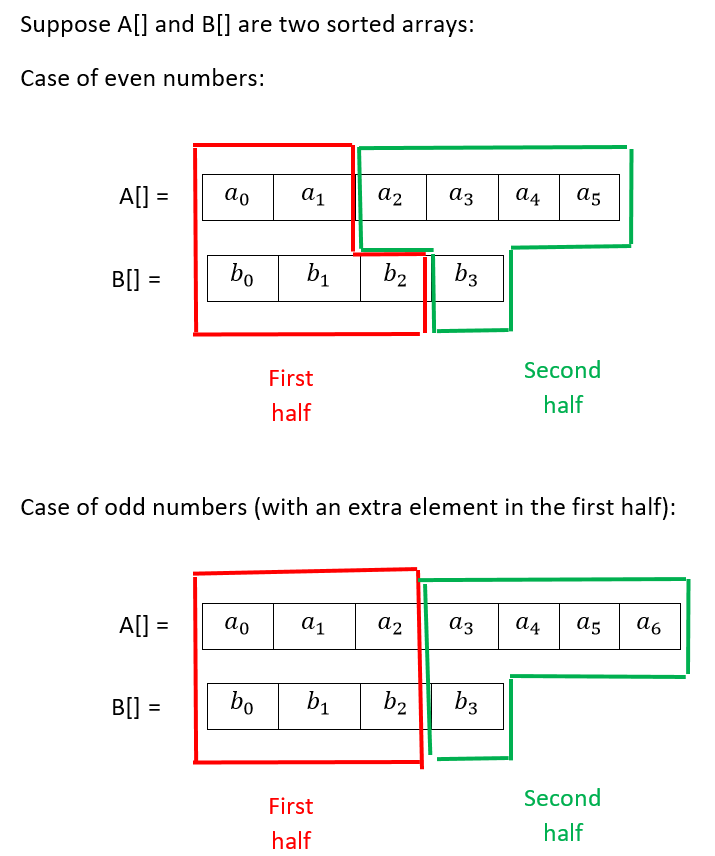Why the above condition leads to the median ?
The median is the (n + 1) / 2 smallest element of the array, and here, the median is the (n + m + 1) / 2 smallest element among the two arrays. If, all the elements in the first half are less than (or equal) to all elements in the second half, in case of odd numbers in total, just calculate the maximum between the last two elements in the first half (a2 and b2 in our example),and this will lead us to the (n + m + 1) / 2 smallest element among the two arrays, which is the median ((7 + 4 + 1) / 2 = 6). But in case of even numbers in total, calculate the average between the maximum of the last two elements in the first half (a1 and b2 in our example) with its successive number among the arrays which is the minimum of first two elements in the second half (a2 and b3 in our example).

The process of the partition :
To make two halves, make the partition such that the index that partitioning array A[] + the index that partitioning array B[] are equal to the total number of elements plus one divided by 2, i.e. (n + m + 1) / 2 (+1 is, if the total number of elements is odd).
First, define two variables : min_index and max_index, and initialize min_index to 0, and max_index to the length of the smaller array. In these below examples A[] is the smaller array.
To partition A[], use the formula (min_index + max_index) / 2 and insert it to a variable i. To partition B[], use the formula (n + m + 1) / 2 – i and insert it to a variable j.
the variable i means the number of elements to be inserted from A[] into the first half, and j means the number of elements to be inserted from B[] into the first half, the rest of the elements will be inserted into the second half.
Take a look at the below examples :

Note: The partition didn’t work if any one array is empty from the given arrays:

For example: if arr1=, arr2=[] by this  “(n + m + 1) / 2” formula the value of i=0 and value of j=1 and this give you out of index error because arr2 is empty and arr2[j] give you out of index error. You have to handle this case by checking if one array is empty you can simply return the medium of another array.

for example:

```if length of arr1 is 0:
return median of arr2
else if length of arr2 is 0:
return median of arr1```

Example 1 :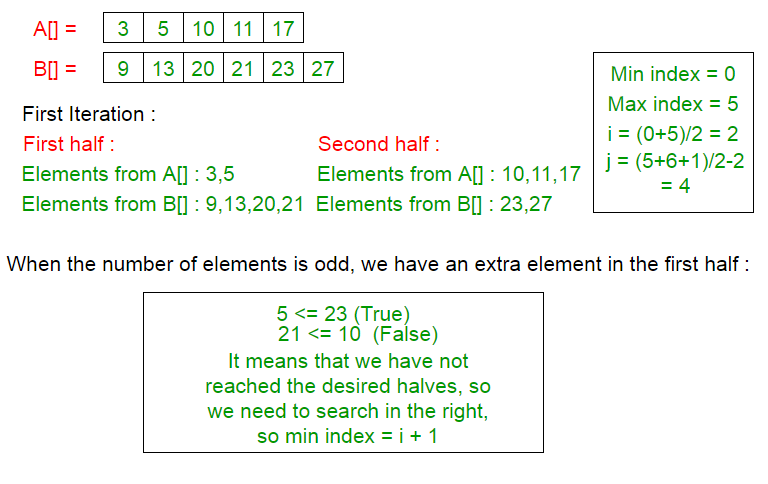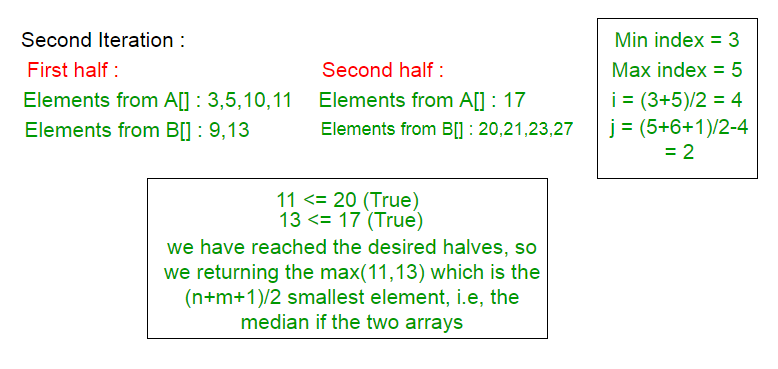Example 2 (This example refers to the condition that returns a median that exists in the merged array) :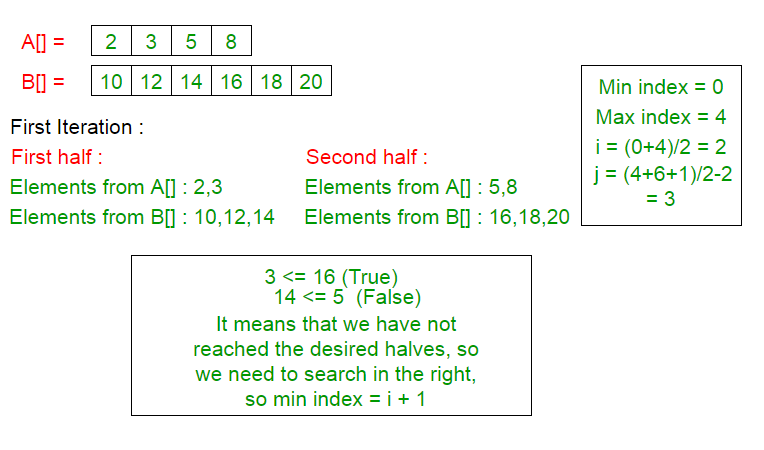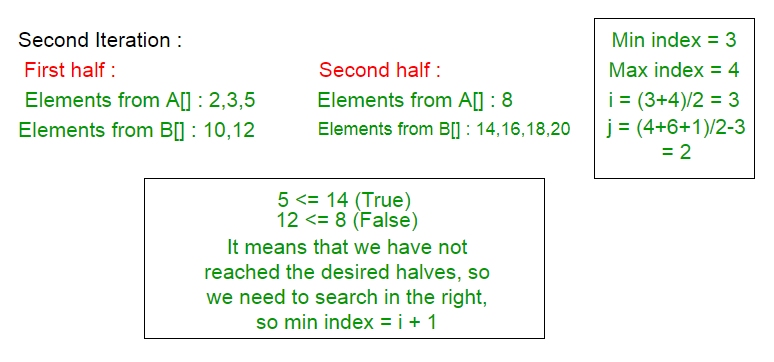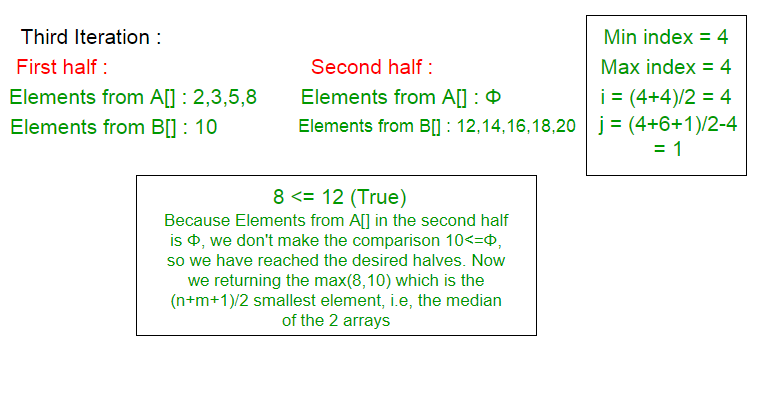Below is the implementation of above approach :

## C++

 `// CPP code for median with case of returning``// double value when even number of elements are``// present in both array combinely``#include``using` `std::cout;` `int` `maximum(``int` `a, ``int` `b);``int` `minimum(``int` `a, ``int` `b);` `// Function to find median of two sorted arrays``double` `findMedianSortedArrays(``int` `*a, ``int` `n,``                              ``int` `*b, ``int` `m)``{``    ` `    ``int` `min_index = 0, max_index = n, i, j, median;``    ` `    ``while` `(min_index <= max_index)``    ``{``        ``i = (min_index + max_index) / 2;``        ``j = ((n + m + 1) / 2) - i;``        ` `        ``// If j is negative then the partition is not``        ``// possible having i elements from array i``        ``if` `(j < 0)``        ``{``            ``max_index = i-1;``            ``continue``;``        ``}` `        ``// if i = n, it means that Elements from a[] in``        ``// the second half is an empty set. and if j = 0,``        ``// it means that Elements from b[] in the first``        ``// half is an empty set. so it is necessary to``        ``// check that, because we compare elements from``        ``// these two groups.``        ``// Searching on right``        ``if` `(i < n && j > 0 && b[j - 1] > a[i])       ``            ``min_index = i + 1;``                ` `        ``// if i = 0, it means that Elements from a[] in``        ``// the first half is an empty set and if j = m,``        ``// it means that Elements from b[] in the second``        ``// half is an empty set. so it is necessary to``        ``// check that, because we compare elements``        ``// from these two groups.``        ``// searching on left``        ``else` `if` `(i > 0 && j < m && b[j] < a[i - 1])       ``            ``max_index = i - 1;` `        ``// we have found the desired halves.``        ``else``        ``{``            ``// this condition happens when we don't have any``            ``// elements in the first half from a[] so we``            ``// returning the last element in b[] from``            ``// the first half.``            ``if` `(i == 0)           ``                ``median = b[j - 1];           ``            ` `            ``// and this condition happens when we don't``            ``// have any elements in the first half from``            ``// b[] so we returning the last element in``            ``// a[] from the first half.``            ``else` `if` `(j == 0)           ``                ``median = a[i - 1];           ``            ``else`           `                ``median = maximum(a[i - 1], b[j - 1]);           ``            ``break``;``        ``}``    ``}``    ` `    ``// calculating the median.``    ``// If number of elements is odd there is``    ``// one middle element.``    ``if` `((n + m) % 2 == 1)``        ``return` `(``double``)median;``        ` `    ``// Elements from a[] in the second half is an empty set.   ``    ``if` `(i == n)``        ``return` `(median+b[j]) / 2.0;``        ` `    ``// Elements from b[] in the second half is an empty set.``    ``if` `(j == m)``        ``return` `(median + a[i]) / 2.0;``    ` `    ``return` `(median + minimum(a[i], b[j])) / 2.0;``}` `// Function to find max``int` `maximum(``int` `a, ``int` `b)``{``    ``return` `a > b ? a : b;``}` `// Function to find minimum``int` `minimum(``int` `a, ``int` `b)``{``    ``return` `a < b ? a : b;``}` `// Driver code``int` `main()``{``    ``int` `a[] = {900};``    ``int` `b[] = { 10, 13, 14};``    ``int` `n = ``sizeof``(a) / ``sizeof``(``int``);``    ``int` `m = ``sizeof``(b) / ``sizeof``(``int``);``    ` `    ``// we need to define the smaller array as the``    ``// first parameter to make sure that the``    ``// time complexity will be O(log(min(n,m)))``    ``if` `(n < m)``        ``cout << ``"The median is : "``             ``<< findMedianSortedArrays(a, n, b, m);``    ``else``        ``cout << ``"The median is : "``             ``<< findMedianSortedArrays(b, m, a, n);` `    ``return` `0;``}`

## Java

 `// Java code for median with``// case of returning double``// value when even number of``// elements are present in``// both array combinely``import` `java.io.*;` `class` `GFG``{``    ``static` `int` `[]a = ``new` `int``[]{``900``};``    ``static` `int` `[]b = ``new` `int``[]{``10``, ``13``, ``14``};` `    ``// Function to find max``    ``static` `int` `maximum(``int` `a, ``int` `b)``    ``{``        ``return` `a > b ? a : b;``    ``}``    ` `    ``// Function to find minimum``    ``static` `int` `minimum(``int` `a, ``int` `b)``    ``{``        ``return` `a < b ? a : b;``    ``}``    ` `    ``// Function to find median``    ``// of two sorted arrays``    ``static` `double` `findMedianSortedArrays(``int` `n,``                                         ``int` `m)``    ``{``        ` `        ``int` `min_index = ``0``,``            ``max_index = n, i = ``0``,``            ``j = ``0``, median = ``0``;``        ` `        ``while` `(min_index <= max_index)``        ``{``            ``i = (min_index + max_index) / ``2``;``            ``j = ((n + m + ``1``) / ``2``) - i;``        ` `            ``// if i = n, it means that Elements``            ``// from a[] in the second half is an``            ``// empty set. and if j = 0, it means``            ``// that Elements from b[] in the first``            ``// half is an empty set. so it is``            ``// necessary to check that, because we``            ``// compare elements from these two``            ``// groups. Searching on right``            ``if` `(i < n && j > ``0` `&& b[j - ``1``] > a[i])    ``                ``min_index = i + ``1``;``                    ` `            ``// if i = 0, it means that Elements``            ``// from a[] in the first half is an``            ``// empty set and if j = m, it means``            ``// that Elements from b[] in the second``            ``// half is an empty set. so it is``            ``// necessary to check that, because``            ``// we compare elements from these two``            ``// groups. searching on left``            ``else` `if` `(i > ``0` `&& j < m && b[j] < a[i - ``1``])    ``                ``max_index = i - ``1``;``    ` `            ``// we have found the desired halves.``            ``else``            ``{``                ``// this condition happens when we``                ``// don't have any elements in the``                ``// first half from a[] so we``                ``// returning the last element in``                ``// b[] from the first half.``                ``if` `(i == ``0``)        ``                    ``median = b[j - ``1``];        ``                ` `                ``// and this condition happens when``                ``// we don't have any elements in the``                ``// first half from b[] so we``                ``// returning the last element in``                ``// a[] from the first half.``                ``else` `if` `(j == ``0``)        ``                    ``median = a[i - ``1``];        ``                ``else`   `                    ``median = maximum(a[i - ``1``],``                                     ``b[j - ``1``]);        ``                ``break``;``            ``}``        ``}``        ` `        ``// calculating the median.``        ``// If number of elements is odd``        ``// there is one middle element.``        ``if` `((n + m) % ``2` `== ``1``)``            ``return` `(``double``)median;``            ` `        ``// Elements from a[] in the``        ``// second half is an empty set.``        ``if` `(i == n)``            ``return` `(median + b[j]) / ``2.0``;``            ` `        ``// Elements from b[] in the``        ``// second half is an empty set.``        ``if` `(j == m)``            ``return` `(median + a[i]) / ``2.0``;``        ` `        ``return` `(median + minimum(a[i],``                                 ``b[j])) / ``2.0``;``    ``}``    ` `    ``// Driver code``    ``public` `static` `void` `main(String args[])``    ``{``        ``int` `n = a.length;``        ``int` `m = b.length;``        ` `        ``// we need to define the``        ``// smaller array as the``        ``// first parameter to``        ``// make sure that the``        ``// time complexity will``        ``// be O(log(min(n,m)))``        ``if` `(n < m)``            ``System.out.print(``"The median is : "` `+``                   ``findMedianSortedArrays(n, m));``        ``else``            ``System.out.print(``"The median is : "` `+``                   ``findMedianSortedArrays(m, n));``    ``}``}` `// This code is contributed by``// Manish Shaw(manishshaw1)`

## Python3

 `# Python code for median with``# case of returning double``# value when even number``# of elements are present``# in both array combinely``median ``=` `0``i ``=` `0``j ``=` `0` `# def to find max`  `def` `maximum(a, b):``    ``return` `a ``if` `a > b ``else` `b` `# def to find minimum`  `def` `minimum(a, b):``    ``return` `a ``if` `a < b ``else` `b` `# def to find median``# of two sorted arrays`  `def` `findMedianSortedArrays(a, n, b, m):` `      ``# if a is empty``      ``if` `n ``=``=` `0``:``      ``# if b has even no. of elements``          ``if` `m ``%` `2` `=``=` `0``:``            ``return` `(b[m``/``2``]``+``b[(m``/``2``)``+``1``])``/``2``      ``# if b has odd no. of elements``          ``else``:``            ``return` `b[``int``(m``/``2``)]` `     ``# if b is empty``      ``elif` `m ``=``=` `0``:``      ``# if a has even no. of elements``           ``if` `n``%``2``=``=``0``:``              ``return` `(a[n``/``2``]``+``a[(n``/``2``)``+``1``])``/``2``      ``# if a has odd no. of elements``      ``else``:``           ``return` `a[``int``(n``/``2``)]` `      ` `global` `median, i, j``min_index ``=` `0``max_index ``=` `n` `while` `(min_index <``=` `max_index):` `        ``i ``=` `int``((min_index ``+` `max_index) ``/` `2``)``        ``j ``=` `int``(((n ``+` `m ``+` `1``) ``/` `2``) ``-` `i)` `        ``# if i = n, it means that``        ``# Elements from a[] in the``        ``# second half is an empty``        ``# set. and if j = 0, it``        ``# means that Elements from``        ``# b[] in the first half is``        ``# an empty set. so it is``        ``# necessary to check that,``        ``# because we compare elements``        ``# from these two groups.``        ``# Searching on right``        ``if` `(i < n ``and` `j > ``0` `and` `b[j ``-` `1``] > a[i]):``            ``min_index ``=` `i ``+` `1` `        ``# if i = 0, it means that``        ``# Elements from a[] in the``        ``# first half is an empty``        ``# set and if j = m, it means``        ``# that Elements from b[] in``        ``# the second half is an empty``        ``# set. so it is necessary to``        ``# check that, because we compare``        ``# elements from these two groups.``        ``# searching on left``        ``elif` `(i > ``0` `and` `j < m ``and` `b[j] < a[i ``-` `1``]):``            ``max_index ``=` `i ``-` `1` `        ``# we have found the``        ``# desired halves.``        ``else``:` `            ``# this condition happens when``            ``# we don't have any elements``            ``# in the first half from a[]``            ``# so we returning the last``            ``# element in b[] from the``            ``# first half.``            ``if` `(i ``=``=` `0``):``                ``median ``=` `b[j ``-` `1``]` `            ``# and this condition happens``            ``# when we don't have any``            ``# elements in the first half``            ``# from b[] so we returning the``            ``# last element in a[] from the``            ``# first half.``            ``elif` `(j ``=``=` `0``):``                ``median ``=` `a[i ``-` `1``]``            ``else``:``                ``median ``=` `maximum(a[i ``-` `1``], b[j ``-` `1``])``            ``break` `    ``# calculating the median.``    ``# If number of elements``    ``# is odd there is``    ``# one middle element.` `    ``if` `((n ``+` `m) ``%` `2` `=``=` `1``):``         ``return` `median` `    ``# Elements from a[] in the``    ``# second half is an empty set.``    ``if` `(i ``=``=` `n):``        ``return` `((median ``+` `b[j]) ``/` `2.0``)` `    ``# Elements from b[] in the``    ``# second half is an empty set.``    ``if` `(j ``=``=` `m):``        ``return` `((median ``+` `a[i]) ``/` `2.0``)` `    ``return` `((median ``+` `minimum(a[i], b[j])) ``/` `2.0``)`  `# Driver code``a ``=` `[``900``]``b ``=` `[``10``, ``13``, ``14``]``n ``=` `len``(a)``m ``=` `len``(b)` `# we need to define the``# smaller array as the``# first parameter to make``# sure that the time complexity``# will be O(log(min(n,m)))``if` `(n < m):``    ``print``(``"The median is : {}"``.``format``(findMedianSortedArrays(a, n, b, m)))``else``:``    ``echo(``"The median is : {}"``.``format``(findMedianSortedArrays(b, m, a, n)))` `# This code is contributed``# by Aditya Khare(adityaskhare123)`

## C#

 `// C# code for median with case of returning``// double value when even number of elements``// are present in both array combinely``using` `System;` `class` `GFG {``    ` `    ``// Function to find max``    ``static` `int` `maximum(``int` `a, ``int` `b)``    ``{``        ``return` `a > b ? a : b;``    ``}``    ` `    ``// Function to find minimum``    ``static` `int` `minimum(``int` `a, ``int` `b)``    ``{``        ``return` `a < b ? a : b;``    ``}``    ` `    ``// Function to find median of two sorted``    ``// arrays``    ``static` `double` `findMedianSortedArrays(``ref` `int` `[]a,``                           ``int` `n, ``ref` `int` `[]b, ``int` `m)``    ``{``        ` `        ``int` `min_index = 0, max_index = n, i = 0,``        ``j = 0, median = 0;``        ` `        ``while` `(min_index <= max_index)``        ``{``            ``i = (min_index + max_index) / 2;``            ``j = ((n + m + 1) / 2) - i;``        ` `            ``// if i = n, it means that Elements``            ``// from a[] in the second half is an``            ``// empty set. and if j = 0, it means``            ``// that Elements from b[] in the first``            ``// half is an empty set. so it is``            ``// necessary to check that, because we``            ``// compare elements from these two``            ``// groups. Searching on right``            ``if` `(i < n && j > 0 && b[j - 1] > a[i])    ``                ``min_index = i + 1;``                    ` `            ``// if i = 0, it means that Elements``            ``// from a[] in the first half is an``            ``// empty set and if j = m, it means``            ``// that Elements from b[] in the second``            ``// half is an empty set. so it is``            ``// necessary to check that, because``            ``// we compare elements from these two``            ``// groups. searching on left``            ``else` `if` `(i > 0 && j < m && b[j] < a[i - 1])    ``                ``max_index = i - 1;``    ` `            ``// we have found the desired halves.``            ``else``            ``{``                ``// this condition happens when we``                ``// don't have any elements in the``                ``// first half from a[] so we``                ``// returning the last element in``                ``// b[] from the first half.``                ``if` `(i == 0)        ``                    ``median = b[j - 1];        ``                ` `                ``// and this condition happens when``                ``// we don't have any elements in the``                ``// first half from b[] so we``                ``// returning the last element in``                ``// a[] from the first half.``                ``else` `if` `(j == 0)        ``                    ``median = a[i - 1];        ``                ``else`       `                    ``median = maximum(a[i - 1], b[j - 1]);        ``                ``break``;``            ``}``        ``}``        ` `        ``// calculating the median.``        ``// If number of elements is odd``        ``// there is one middle element.``        ``if` `((n + m) % 2 == 1)``            ``return` `(``double``)median;``            ` `        ``// Elements from a[] in the second``        ``// half is an empty set.``        ``if` `(i == n)``            ``return` `(median+b[j]) / 2.0;``            ` `        ``// Elements from b[] in the second``        ``// half is an empty set.``        ``if` `(j == m)``            ``return` `(median + a[i]) / 2.0;``        ` `        ``return` `(median + minimum(a[i], b[j])) / 2.0;``    ``}``    ` `    ``// Driver code``    ``static` `void` `Main()``    ``{``        ``int` `[]a = ``new` `int``[]{900};``        ``int` `[]b = ``new` `int``[]{ 10, 13, 14};``        ``int` `n = a.Length;``        ``int` `m = b.Length;``        ` `        ``// we need to define the smaller``        ``// array as the first parameter to``        ``// make sure that the time``        ``// complexity will be O(log(min(n,m)))``        ``if` `(n < m)``            ``Console.Write(``"The median is : "``            ``+ findMedianSortedArrays(``ref` `a, n,``                                   ``ref` `b, m));``        ``else``            ``Console.Write(``"The median is : "``            ``+ findMedianSortedArrays(``ref` `b, m,``                                   ``ref` `a, n));``    ``}``}` `// This code is contributed by Manish Shaw``// (manishshaw1)`

## PHP

 ` ``\$b` `? ``\$a` `: ``\$b``;``}` `// Function to find minimum``function` `minimum(``\$a``, ``\$b``)``{``    ``return` `\$a` `< ``\$b` `? ``\$a` `: ``\$b``;``}` `// Function to find median``// of two sorted arrays``function` `findMedianSortedArrays(&``\$a``, ``\$n``,``                                ``&``\$b``, ``\$m``)``{``    ``global` `\$median``, ``\$i``, ``\$j``;``    ``\$min_index` `= 0;``    ``\$max_index` `= ``\$n``;``    ` `    ``while` `(``\$min_index` `<= ``\$max_index``)``    ``{``        ``\$i` `= ``intval``((``\$min_index` `+``                     ``\$max_index``) / 2);``        ``\$j` `= ``intval``(((``\$n` `+ ``\$m` `+ 1) /``                           ``2) - ``\$i``);``    ` `        ``// if i = n, it means that``        ``// Elements from a[] in the``        ``// second half is an empty``        ``// set. and if j = 0, it``        ``// means that Elements from``        ``// b[] in the first half is``        ``// an empty set. so it is``        ``// necessary to check that,``        ``// because we compare elements``        ``// from these two groups.``        ``// Searching on right``        ``if` `(``\$i` `< ``\$n` `&& ``\$j` `> 0 &&``            ``\$b``[``\$j` `- 1] > ``\$a``[``\$i``])    ``            ``\$min_index` `= ``\$i` `+ 1;``                ` `        ``// if i = 0, it means that``        ``// Elements from a[] in the``        ``// first half is an empty``        ``// set and if j = m, it means``        ``// that Elements from b[] in``        ``// the second half is an empty``        ``// set. so it is necessary to``        ``// check that, because we compare``        ``// elements from these two groups.``        ``// searching on left``        ``else` `if` `(``\$i` `> 0 && ``\$j` `< ``\$m` `&&``                 ``\$b``[``\$j``] < ``\$a``[``\$i` `- 1])    ``            ``\$max_index` `= ``\$i` `- 1;``        ` `        ``// we have found the``        ``// desired halves.``        ``else``        ``{``            ``// this condition happens when``            ``// we don't have any elements``            ``// in the first half from a[]``            ``// so we returning the last``            ``// element in b[] from the``            ``// first half.``            ``if` `(``\$i` `== 0)``                ``\$median` `= ``\$b``[``\$j` `- 1];``                ` `            ``// and this condition happens``            ``// when we don't have any``            ``// elements in the first half``            ``// from b[] so we returning the``            ``// last element in a[] from the``            ``// first half.``            ``else` `if` `(``\$j` `== 0)        ``                ``\$median` `= ``\$a``[``\$i` `- 1];        ``            ``else`       `                ``\$median` `= maximum(``\$a``[``\$i` `- 1],``                                  ``\$b``[``\$j` `- 1]);``            ``break``;``        ``}``    ``}``    ` `    ``// calculating the median.``    ``// If number of elements``    ``// is odd there is``    ``// one middle element.` `    ``if` `((``\$n` `+ ``\$m``) % 2 == 1)``        ``return` `\$median``;` `    ``// Elements from a[] in the``    ``// second half is an empty set.``    ``if` `(``\$i` `== ``\$n``)``        ``return` `((``\$median` `+``                 ``\$b``[``\$j``]) / 2.0);` `    ``// Elements from b[] in the``    ``// second half is an empty set.``    ``if` `(``\$j` `== ``\$m``)``        ``return` `((``\$median` `+``                 ``\$a``[``\$i``]) / 2.0);``    ` `    ``return` `((``\$median` `+``             ``minimum(``\$a``[``\$i``],``                     ``\$b``[``\$j``])) / 2.0);``}` `// Driver code``\$a` `= ``array``(900);``\$b` `= ``array``(10, 13, 14);``\$n` `= ``count``(``\$a``);``\$m` `= ``count``(``\$b``);` `// we need to define the``// smaller array as the``// first parameter to make``// sure that the time complexity``// will be O(log(min(n,m)))``if` `(``\$n` `< ``\$m``)``    ``echo` `(``"The median is : "` `.``           ``findMedianSortedArrays(``\$a``, ``\$n``,``                                  ``\$b``, ``\$m``));``else``    ``echo` `(``"The median is : "` `.``           ``findMedianSortedArrays(``\$b``, ``\$m``,``                                  ``\$a``, ``\$n``));``                                  ` `// This code is contributed``// by Manish Shaw(manishshaw1)``?>`

## Javascript

 ``

Output:

`The median is : 13.5`

Time Complexity : O(log(min(n, m)),where n is the size of the first array and m is the size of the second array. This is because we are using binary search to find the median. We are dividing the array in halves in each iteration, so the time complexity will be O(log(min(n, m))).
Space Complexity : O(1),the space complexity of this algorithm is O(1), as we are not using any extra space.

Another Approach: Same program, but returns the median that exists in the merged array((n + m) / 2 – 1 position):

Implementation:

## C++

 `// C++ code for finding median of the given two``// sorted arrays that exists in the merged array ((n+m) / 2 - 1 position)``#include``using` `std::cout;` `int` `maximum(``int` `a, ``int` `b);` `// Function to find median of given two sorted arrays``int` `findMedianSortedArrays(``int` `*a, ``int` `n,``                           ``int` `*b, ``int` `m)``{``    ` `    ``int` `min_index = 0, max_index = n, i, j;``    ` `    ``while` `(min_index <= max_index)``    ``{``        ``i = (min_index + max_index) / 2;``        ``j = ((n + m + 1) / 2) - i;``    ` `        ``// if i = n, it means that Elements from a[] in``        ``// the second half is an empty set. If j = 0, it``        ``// means that Elements from b[] in the first half``        ``// is an empty set. so it is necessary to check that,``        ``// because we compare elements from these two groups.``        ``// searching on right``        ``if` `(i < n && j > 0 && b[j - 1] > a[i])       ``            ``min_index = i + 1;       ``        ` `        ``// if i = 0, it means that Elements from a[] in the``        ``// first half is an empty set and if j = m, it means``        ``// that Elements from b[] in the second half is an``        ``// empty set. so it is necessary to check that,``        ``// because we compare elements from these two groups.``        ``// searching on left``        ``else` `if` `(i > 0 && j < m && b[j] < a[i - 1])       ``            ``max_index = i - 1;       ``        ` `        ``// we have found the desired halves.``        ``else``        ``{``            ``// this condition happens when we don't have``            ``// any elements in the first half from a[] so``            ``// we returning the last element in b[] from``            ``// the first half.``            ``if` `(i == 0)           ``                ``return` `b[j - 1];           ``            ` `            ``// and this condition happens when we don't have any``            ``// elements in the first half from b[] so we``            ``// returning the last element in a[] from the first half.``            ``if` `(j == 0)           ``                ``return` `a[i - 1];           ``            ``else`           `                ``return` `maximum(a[i - 1], b[j - 1]);          ``        ``}``    ``}``    ` `    ``cout << ``"ERROR!!! "` `<< ``"returning\n"``;   ``    ``return` `0;``}` `// Function to find maximum``int` `maximum(``int` `a, ``int` `b)``{``    ``return` `a > b ? a : b;``}` `// Driver code``int` `main()``{``    ``int` `a[] = {900};``    ``int` `b[] = { 10,13,14};``    ``int` `n = ``sizeof``(a) / ``sizeof``(``int``);``    ``int` `m = ``sizeof``(b) / ``sizeof``(``int``);``    ` `    ``// we need to define the smaller array as the first``    ``// parameter to make sure that the time complexity``    ``// will be O(log(min(n,m)))``    ``if` `(n < m)``        ``cout << ``"The median is: "``             ``<< findMedianSortedArrays(a, n, b, m);``    ``else``        ``cout << ``"The median is: "``             ``<< findMedianSortedArrays(b, m, a, n);``    ``return` `0;``}`

## Java

 `// Java code for finding median of the``// given two sorted arrays that exists``// in the merged array ((n+m) / 2 -``// 1 position)``import` `java.io.*;` `class` `GFG{``    ` `// Function to find maximum``static` `int` `maximum(``int` `a, ``int` `b) ``{``    ``return` `a > b ? a : b; ``}     ``  ` `// Function to find median ``// of given two sorted arrays``static` `int` `findMedianSortedArrays(``int` `[]a, ``int` `n, ``                                  ``int` `[]b, ``int` `m)``{``    ``int` `min_index = ``0``, ``        ``max_index = n, i, j;``      ` `    ``while` `(min_index <= max_index)``    ``{``        ``i = (min_index + max_index) / ``2``;``        ``j = ((n + m + ``1``) / ``2``) - i;``      ` `        ``// If i = n, it means that ``        ``// Elements from a[] in the ``        ``// second half is an empty ``        ``// set. If j = 0, it means ``        ``// that Elements from b[] ``        ``// in the first half is an ``        ``// empty set. so it is ``        ``// necessary to check that,``        ``// because we compare elements ``        ``// from these two groups. ``        ``// searching on right``        ``if` `(i < n && j > ``0` `&& ``            ``b[j - ``1``] > a[i])     ``            ``min_index = i + ``1``;     ``          ` `        ``// If i = 0, it means that ``        ``// Elements from a[] in the``        ``// first half is an empty set ``        ``// and if j = m, it means that``        ``// Elements from b[] in the ``        ``// second half is an empty set.``        ``// so it is necessary to check ``        ``// that, because we compare ``        ``// elements from these two ``        ``// groups. searching on left``        ``else` `if` `(i > ``0` `&& j < m && ``                 ``b[j] < a[i - ``1``])     ``            ``max_index = i - ``1``;     ``          ` `        ``// We have found the``        ``// desired halves.``        ``else``        ``{``            ` `            ``// This condition happens ``            ``// when we don't have any ``            ``// elements in the first ``            ``// half from a[] so we ``            ``// returning the last ``            ``// element in b[] from``            ``// the first half.``            ``if` `(i == ``0``)         ``                ``return` `b[j - ``1``];         ``              ` `            ``// And this condition happens ``            ``// when we don't have any ``            ``// elements in the first half ``            ``// from b[] so we returning the``            ``// last element in a[] from the``            ``// first half.``            ``if` `(j == ``0``)         ``                ``return` `a[i - ``1``];         ``            ``else`       `                ``return` `maximum(a[i - ``1``],``                               ``b[j - ``1``]);         ``        ``}``    ``}``      ` `    ``System.out.print(``"ERROR!!! "` `+ ``                     ``"returning\n"``); ``    ``return` `0``;``}     ``  ` `// Driver code``public` `static` `void` `main(String[] args)``{``    ``int` `[]a = {``900``};``    ``int` `[]b = {``10``, ``13``, ``14``};``    ``int` `n = a.length;``    ``int` `m = b.length;``      ` `    ``// We need to define the smaller ``    ``// array as the first parameter ``    ``// to make sure that the time ``    ``// complexity will be O(log(min(n,m)))``    ``if` `(n < m)``        ``System.out.print(``"The median is: "` `+``                         ``findMedianSortedArrays(a, n, ``                                                ``b, m));``    ``else``        ``System.out.print(``"The median is: "` `+ ``                         ``findMedianSortedArrays(b, m, ``                                                ``a, n));``}``}` `// This code is contributed by rag2127`

## Python3

 `# Python 3 code for finding median``# of the given two sorted arrays that``# exists in the merged array``# ((n+m) / 2 - 1 position)` `# Function to find median of given``# two sorted arrays``def` `findMedianSortedArrays(a, n, b, m):``    ` `    ``min_index ``=` `0``    ``max_index ``=` `n``    ` `    ``while` `(min_index <``=` `max_index):``        ` `        ``i ``=` `(min_index ``+` `max_index) ``/``/` `2``        ``j ``=` `((n ``+` `m ``+` `1``) ``/``/` `2``) ``-` `i``    ` `        ``# if i = n, it means that Elements``        ``# from a[] in the second half is``        ``# an empty set. If j = 0, it means``        ``# that Elements from b[] in the first``        ``# half is an empty set. so it is necessary``        ``# to check that, because we compare elements``        ``# from these two groups. searching on right``        ``if` `(i < n ``and` `j > ``0` `and` `b[j ``-` `1``] > a[i]):    ``            ``min_index ``=` `i ``+` `1`   `        ` `        ``# if i = 0, it means that Elements from``        ``# a[] in the first half is an empty set``        ``# and if j = m, it means that Elements``        ``# from b[] in the second half is an``        ``# a[] in the first half is an empty set ``        ``# that, because we compare elements from``        ``# these two groups. searching on left``        ``elif` `(i > ``0` `and` `j < m ``and` `b[j] < a[i ``-` `1``]):    ``            ``max_index ``=` `i ``-` `1`   `        ` `        ``# we have found the desired halves.``        ``else``:``            ` `            ``# this condition happens when we don't have``            ``# any elements in the first half from a[] so``            ``# we returning the last element in b[] from``            ``# the first half.``            ``if` `(i ``=``=` `0``):``                ``return` `b[j ``-` `1``]        ``            ` `            ``# and this condition happens when we don't``            ``# have any elements in the first half from``            ``# b[] so we returning the last element in``            ``# a[] from the first half.``            ``if` `(j ``=``=` `0``):``                ``return` `a[i ``-` `1``]        ``            ``else``:``                ``return` `maximum(a[i ``-` `1``], b[j ``-` `1``])``    ` `    ``print``(``"ERROR!!! "` `, ``"returning\n"``)``    ``return` `0` `# Function to find maximum``def` `maximum(a, b) :``    ``return` `a ``if` `a > b ``else` `b` `# Driver code``if` `__name__ ``=``=` `"__main__"``:``    ``a ``=` `[``900``]``    ``b ``=` `[``10``, ``13``, ``14``]``    ``n ``=` `len``(a)``    ``m ``=` `len``(b)``    ` `    ``# we need to define the smaller array``    ``# as the first parameter to make sure``    ``# that the time complexity will be``    ``# O(log(min(n,m)))``    ``if` `(n < m):``        ``print``( ``"The median is: "``,  ``                ``findMedianSortedArrays(a, n, b, m))``    ``else``:``        ``print``( ``"The median is: "``,``                ``findMedianSortedArrays(b, m, a, n))` `# This code is contributed``# by ChitraNayal`

## C#

 `// C# code for finding median``// of the given two sorted``// arrays that exists in the``// merged array ((n+m) / 2 -``// 1 position)``using` `System;` `class` `GFG``{``    ``// Function to find maximum``    ``static` `int` `maximum(``int` `a,``                       ``int` `b)``    ``{``        ``return` `a > b ? a : b;``    ``}    ``    ` `    ``// Function to find median``    ``// of given two sorted arrays``    ``static` `int` `findMedianSortedArrays(``ref` `int` `[]a, ``int` `n,``                                      ``ref` `int` `[]b, ``int` `m)``    ``{``        ` `        ``int` `min_index = 0,``            ``max_index = n, i, j;``        ` `        ``while` `(min_index <= max_index)``        ``{``            ``i = (min_index + max_index) / 2;``            ``j = ((n + m + 1) / 2) - i;``        ` `            ``// if i = n, it means that``            ``// Elements from a[] in the``            ``// second half is an empty``            ``// set. If j = 0, it means``            ``// that Elements from b[]``            ``// in the first half is an``            ``// empty set. so it is``            ``// necessary to check that,``            ``// because we compare elements``            ``// from these two groups.``            ``// searching on right``            ``if` `(i < n && j > 0 &&``                ``b[j - 1] > a[i])    ``                ``min_index = i + 1;    ``            ` `            ``// if i = 0, it means that``            ``// Elements from a[] in the``            ``// first half is an empty set``            ``// and if j = m, it means that``            ``// Elements from b[] in the``            ``// second half is an empty set.``            ``// so it is necessary to check``            ``// that, because we compare``            ``// elements from these two``            ``// groups. searching on left``            ``else` `if` `(i > 0 && j < m &&``                     ``b[j] < a[i - 1])    ``                ``max_index = i - 1;    ``            ` `            ``// we have found the``            ``// desired halves.``            ``else``            ``{``                ``// this condition happens``                ``// when we don't have any``                ``// elements in the first``                ``// half from a[] so we``                ``// returning the last``                ``// element in b[] from``                ``// the first half.``                ``if` `(i == 0)        ``                    ``return` `b[j - 1];        ``                ` `                ``// and this condition happens``                ``// when we don't have any``                ``// elements in the first half``                ``// from b[] so we returning the``                ``// last element in a[] from the``                ``// first half.``                ``if` `(j == 0)        ``                    ``return` `a[i - 1];        ``                ``else`       `                    ``return` `maximum(a[i - 1],``                                   ``b[j - 1]);        ``            ``}``        ``}``        ` `        ``Console.Write (``"ERROR!!! "` `+``                       ``"returning\n"``);``        ``return` `0;``    ``}    ``    ` `    ``// Driver code``    ``static` `void` `Main()``    ``{``        ``int` `[]a = ``new` `int``[]{900};``        ``int` `[]b = ``new` `int``[]{10, 13, 14};``        ``int` `n = a.Length;``        ``int` `m = b.Length;``        ` `        ``// we need to define the smaller``        ``// array as the first parameter``        ``// to make sure that the time``        ``// complexity will be O(log(min(n,m)))``        ``if` `(n < m)``            ``Console.Write (``"The median is: "` `+``                            ``findMedianSortedArrays(``ref` `a, n,``                                                   ``ref` `b, m));``        ``else``            ``Console.Write (``"The median is: "` `+``                            ``findMedianSortedArrays(``ref` `b, m,``                                                   ``ref` `a, n));``    ``}``}` `// This code is contributed by``// Manish Shaw(manishshaw1)`

## Javascript

 ``

Output:

`The median is: 13`

Time Complexity: O(log(min(n, m))), where n and m are the sizes of the given sorted array
Auxiliary Space: O(1), as no extra space is used in this algorithm, the space complexity is O(1).

My Personal Notes arrow_drop_up